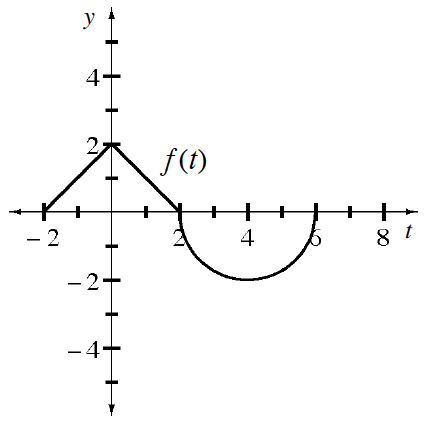### Home > APCALC > Chapter 8 > Lesson 8.2.3 > Problem8-88

8-88.

Let $g(x)=\int_0^xf(t)dt$ where $y = f (t)$ is shown at right.

1. Evaluate $g(2)$ and $g(4)$.

$\text{Since }g(x)\text{ is defined as }\int_{0}^{x}f(t)dt:$

$g(2)=\int_{0}^{2}f(t)dt$

$g(4)=\int_{0}^{4}f(t)dt$

2. Is $g ( - 2 ) = \int _ { - 2 } ^ { 0 } f ( t ) d t$?

Consider the symmetry of $y = f(t)$ between $t = −2$ and $t = 2$.

3. Express $\int _ { - 2 } ^ { 2 } f ( t ) d t$ in terms of $g$.

$\int_{-2}^{2}f(t)dt=kg(a)$

What is $k$? What is $a$?

4. Is $g$ differentiable over the interval $−2 < x < 6$ ? Explain.

Points of NON-differentiability include cusps, endpoints, jumps, holes, and vertical tangents.

5. Determine all values of $x$ in the interval $−2 < x < 6$ where $g$ has a relative maximum.

Recall that a local maximum exists where the derivative changes from positive to negative. This can happen where $f^\prime(x) = 0$ or where $f^\prime(x) = \text{DNE}$.

Notice that $g^\prime(x) = f(x)$; after all, the derivative of an integral is the original function.

6. Write the equation of the line tangent to $g$ at $x = 4$.

What is the slope of $g(x)$ at $x = 4$ (see second hint in part (e))? What is the $y$-value?

7. Determine all values of $x$ in the interval $−2 < x < 6$ where $g$ has a point of inflection.

Concavity is the slope of the slope. So inflection points are where the slope of the slope changes.